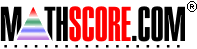Math Practice Online > free > lessons > Florida > 6th grade > Percentages

## Percentages

Calculate percentage given a decimal or fraction.

 Sample Problems for Percentages Lesson for Percentages

### This topic aligns to the following state standards

Grade 5: Num 1. Knows that numbers in different forms are equivalent or nonequivalent, using whole numbers, decimals, fractions, mixed numbers, and percents.
Grade 6: Num 4. Converts a number expressed in one form to its equivalent in another form.
Grade 7: Num 4. Converts a number expressed in one form to its equivalent in another form.
Grade 9: Num 4. understands that numbers can be represented in a variety of equivalent forms, including integers, fractions, decimals, percents, scientific notation, exponents, radicals, absolute value, and logarithms

Copyright Accurate Learning Systems Corporation 2008.